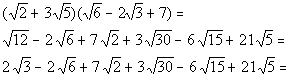# Intermediate Algebra Tutorial 40

Intermediate Algebra
Tutorial 40: Adding, Subtracting and MultiplyingAnswer/Discussion to 1a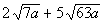The 63 in the second radical has a factor that we can take the square root of.

Can you think of what that factor is?

Let's see what we get when we simplify the second radical: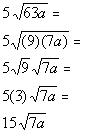*Rewrite 63a as (9)(7a)
*Square root of 9 is 3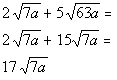Answer/Discussion to 1b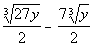We can take the cube root of the 27 in the first radical: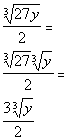*Cube root of 27 is 3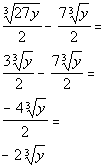Answer/Discussion to 2a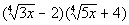Step 1: Multiply the radical expression

AND

We can apply the FOIL method found in Tutorial 26 (Multiplying Polynomials) to this example: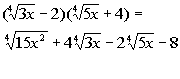Answer/Discussion to 2b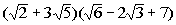Step 1: Multiply the radical expression

AND

Using distributive property twice we get: